# Highlight a row in Excel based on a cell value

In this blog entry we take a look how to highlight a row in a Microsoft Excel table based on a cell value.  First we take a look at the steps required and then we take an example to see it in practice.   We will use the “Conditional Formatting” feature of Microsoft Excel.

Steps to highlight a row in Microsoft Excel:

1. Select the data (table) you want to apply the highlight to.
2. Go to the “Conditional Formatting” option and select “New Rule”.
3. Select the “Use a formula to determine which cells to format”.
4. Put in the formula: =INDIRECT(“<column>”&ROW())=<value>.  Where column is the column of which the value is to be compared, and value is the value to compare to.
5. Click on the “Format” button to specify how you want the row highlighted.
6. Click “Ok”.

## Example

Let’s take a look how this is done in practice. For this example we will use a table with Projected and Actual costs for Project X.  We want to highlight the row that indicates the cost of the current week.  A sample table is show in Figure 1.

### Step 1

Select the table you want to format: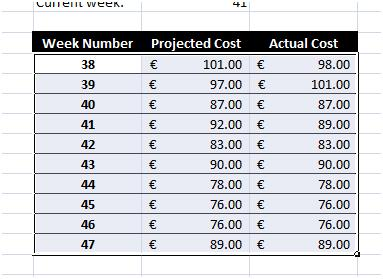Figure 2: Table selection

### Step 2

Go to the “Conditional Formatting” option and select “New Rule”.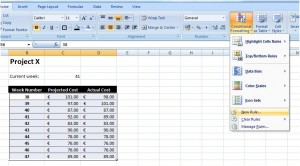Figure 3: Selecting “New Rule...” from the “Conditional Formatting” option

### Step 3

Select the “Use a formula to determine which cells to format”.

### Step 4

Put in the formula: =INDIRECT(“B”&ROW())=\$C\$3. See Figure 4. Note that “B” is the column we want to compare and cell \$C\$3 contains the value we want to compare to.  The value of this cell is automatically updated every week with the week number.  To do this we use the formula =WEEKNUM(NOW(),2) as indicated in Figure 5.  If for example, you want to highlight all the rows that have a future week number, that is, all those whose week number is greater than the current week number, you will replace the equal comparison (=) to a greater than (>) comparison.  Then, the formula will read =INDIRECT(“B”&ROW())>\$C\$3.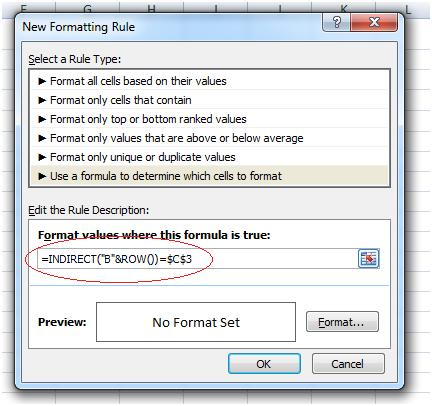Figure 4: Typing in the formula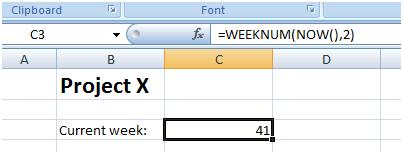Figure 5: Formula to display the current week’s number

### Step 5

The final step is to select the way you want to format your row.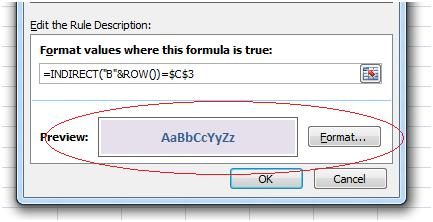Figure 6: Row formatting

### Step 6

When you are satisfied with the formatting, hit the OK button and you are done.  Figure 7 shows the final result.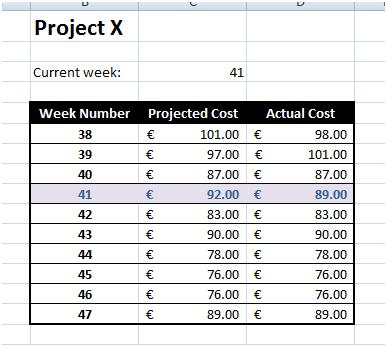Figure 7: Final result